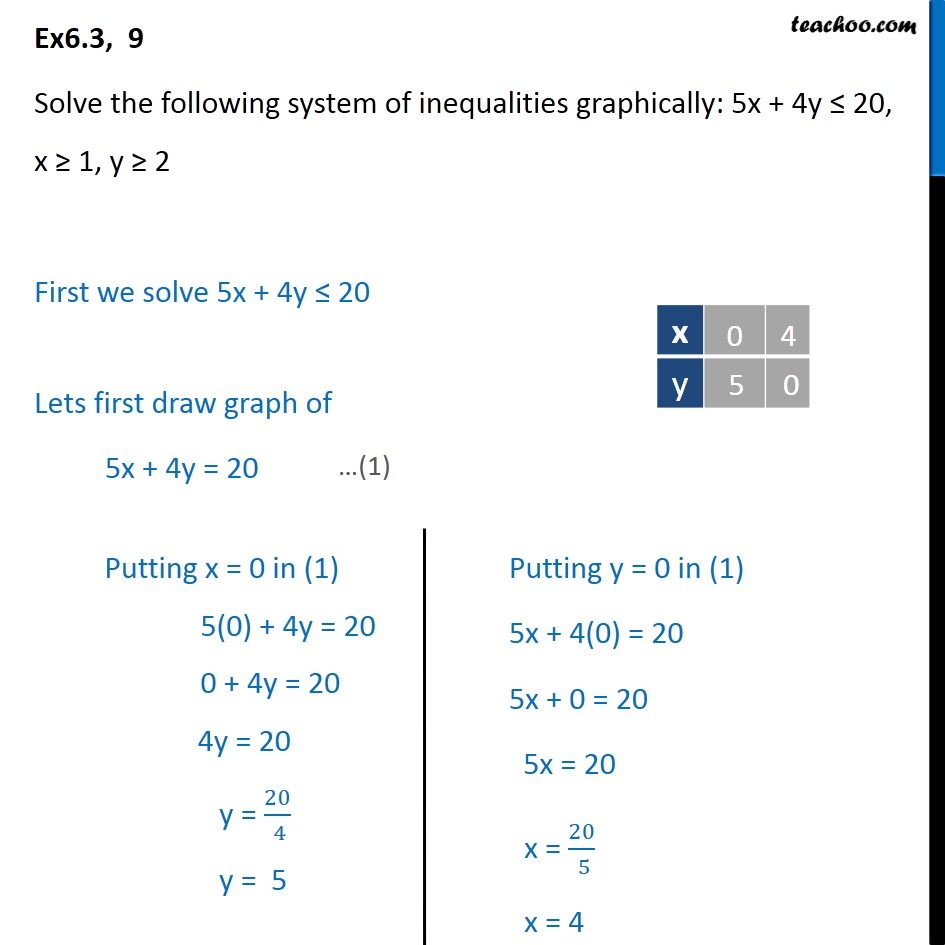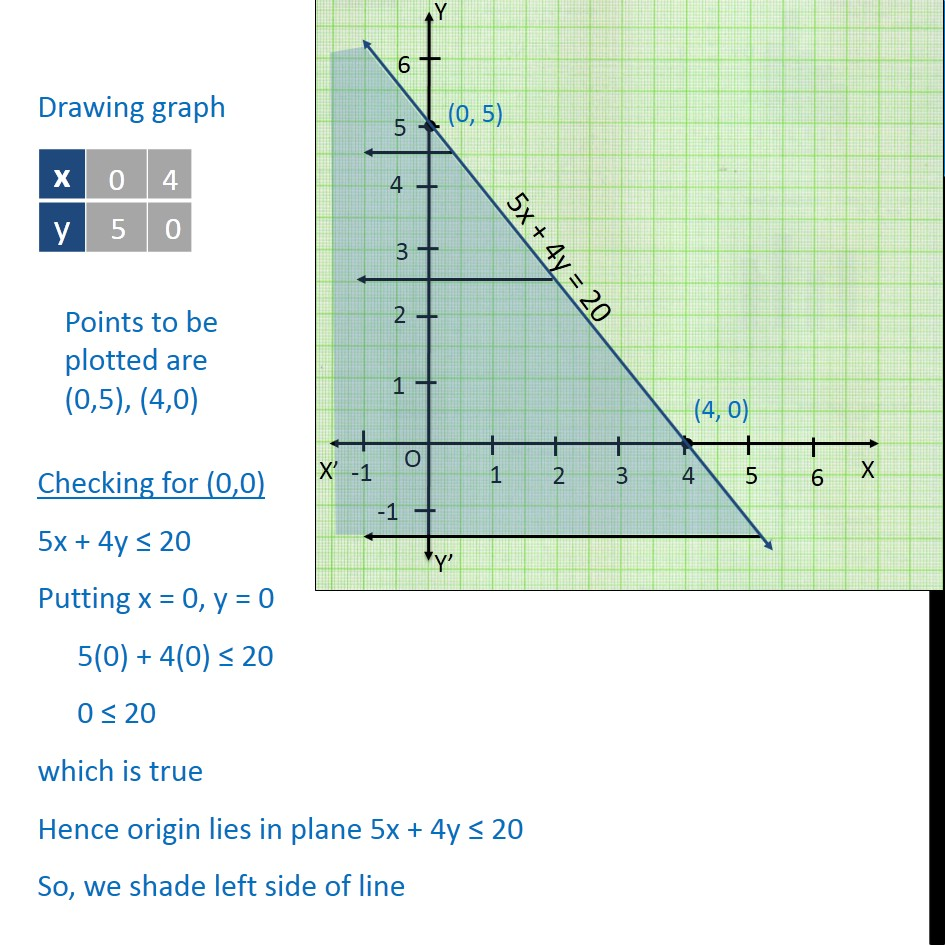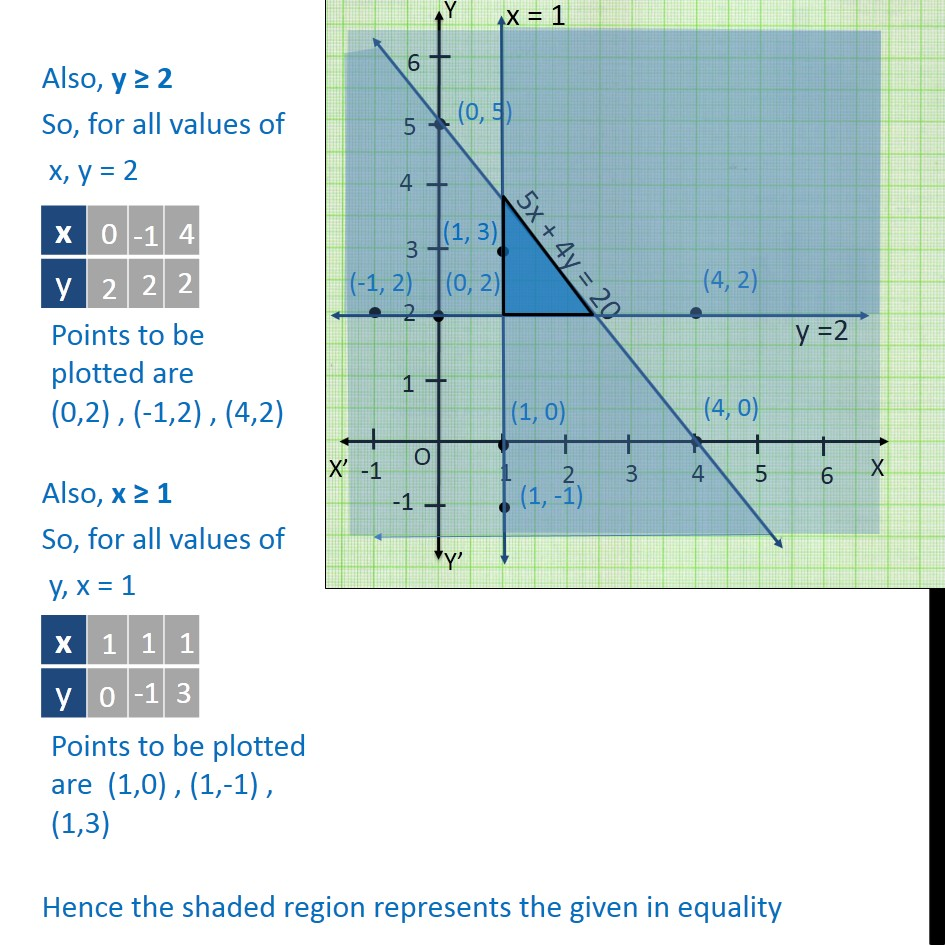Solving Pair of Linear Inequalities

Chapter 5 Class 11 Linear Inequalities
Serial order wiseLearn in your speed, with individual attention - Teachoo Maths 1-on-1 Class

### Transcript

Ex6.3, 9 Solve the following system of inequalities graphically: 5x + 4y 20, x 1, y 2 First we solve 5x + 4y 20 Lets first draw graph of 5x + 4y = 20 Drawing graph Checking for (0,0) 5x + 4y 20 Putting x = 0, y = 0 5(0) + 4(0) 20 0 20 which is true Hence origin lies in plane 5x + 4y 20 So, we shade left side of line Also, y 2 So, for all values of x, y = 2 Also, x 1 So, for all values of y, x = 1 Hence the shaded region represents the given in equality